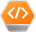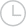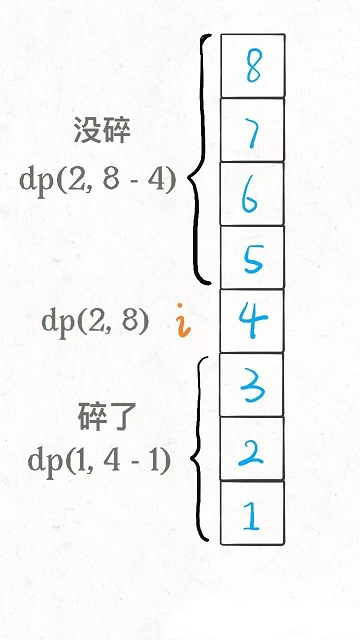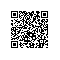# 经典动态规划：高楼扔鸡蛋 6月2日 【今日算法】-问答-阿里云开发者社区-阿里云

## 经典动态规划：高楼扔鸡蛋 6月2日 【今日算法】游客ih62co2qqq5ww2020-06-02 16:06:52889

### 一、解析题目

PS：F 可以为 0，比如说鸡蛋在 1 层都能摔碎，那么 F = 0。

PS：这有点像 Big O 表示法计算算法的复杂度。

### 二、思路分析

「状态」很明显，就是当前拥有的鸡蛋数`K`和需要测试的楼层数`N`。随着测试的进行，鸡蛋个数可能减少，楼层的搜索范围会减小，这就是状态的变化。

「选择」其实就是去选择哪层楼扔鸡蛋。回顾刚才的线性扫描和二分思路，二分查找每次选择到楼层区间的中间去扔鸡蛋，而线性扫描选择一层层向上测试。不同的选择会造成状态的转移。

``````# 当前状态为 (K 个鸡蛋，N 层楼)
# 返回这个状态下的最优结果
def dp(K, N):
int res
for 1 <= i <= N:
res = min(res, 这次在第 i 层楼扔鸡蛋)
return res
``````PS：细心的读者可能会问，在第`i`层楼扔鸡蛋如果没碎，楼层的搜索区间缩小至上面的楼层，是不是应该包含第`i`层楼呀？不必，因为已经包含了。开头说了 F 是可以等于 0 的，向上递归后，第`i`层楼其实就相当于第 0 层，可以被取到，所以说并没有错误。

``````def dp(K, N):
for 1 <= i <= N:
# 最坏情况下的最少扔鸡蛋次数
res = min(res,
max(
dp(K - 1, i - 1), # 碎
dp(K, N - i)      # 没碎
) + 1 # 在第 i 楼扔了一次
)
return res
``````

``````def dp(K, N):
if K == 1: return N
if N == 0: return 0
...
``````

``````def superEggDrop(K: int, N: int):

memo = dict()
def dp(K, N) -> int:
# base case
if K == 1: return N
if N == 0: return 0
# 避免重复计算
if (K, N) in memo:
return memo[(K, N)]

res = float('INF')
# 穷举所有可能的选择
for i in range(1, N + 1):
res = min(res,
max(
dp(K, N - i),
dp(K - 1, i - 1)
) + 1
)
# 记入备忘录
memo[(K, N)] = res
return res

return dp(K, N)
``````

### 三、疑难解答取消 提交回答使用钉钉扫一扫加入圈子
+ 订阅